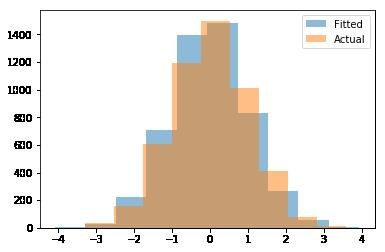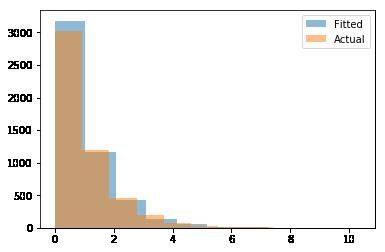Fitting Probability Distributions with Python Part 1

Probability distributions are a powerful tool to use when modeling random processes. They are widely used in statistics, simulations, engineering and various other settings. I have had to use them in various projects to correctly model randomness.

There are many probability distributions to choose, from the well-known normal distribution to many others such as logistic and Weibull. The common problem I have continuously faced is having an easy to use tool to quickly fit the best distribution to my data and then use the best fit distribution to generate random numbers. Once again Python shows its flexibility for data science with its SciPy package, one of the main Python packages for mathematics, science and engineering. We will be using the SciPy package to tackle this task.

The explanation of probability distributions is beyond the scope of this post, if you are unfamiliar with probability distributions I recommend you read up on them first. If you know what probability distributions are and usually need to model them this code will come in handy.

Our Objective

The following python class will allow you to easily fit a continuous distribution to your data. Once the fit has been completed, this python class allows you to then generate random numbers based on the distribution that best fit your data. It contains a variable and P-Value for you to see which distribution it picked. It also has the flexibility for you to define the list of distributions to try and fit your data.

As a mentioned previously, this only works for continuous distributions. I am planning on later adding functionality that allows this to work for discrete distributions. The limitation is that discrete distributions in SciPy don’t have a method to fit the data so we have to implement the fitting in a different manner.

The Code

Let’s start by importing the required packages. As previously mentioned, we will be using the SciPy package and the Matplotlib package for visualizations. This code has been tested in Python 3.4, SciPy Version 0.18.1 and Matplotlib Version 1.5.1.

import scipy
import scipy.stats
import matplotlib
import matplotlib.pyplot as plt

Next, we define our class which we will call Distribution. The initializer accepts a list of distribution names which are implemented in SciPy. If none are provided, the default distributions to fit will be the Normal, Lognormal, Exponential and Pareto distributions. Additional required parameters are also initialized which we will be using later.

class Distribution(object):

def __init__(self,dist_names_list = []):
self.dist_names = ['norm','lognorm','expon','pareto']
self.dist_results = []
self.params = {}

self.DistributionName = ""
self.PValue = 0
self.Param = None

self.isFitted = False

To determine how good of a fit this distribution is, we will use the Kolmogorov-Smirnov test for goodness of fit. This is a nonparametric test to compare a sample with a reference probability distribution. Our sample in this case is our y variable, and our recently fitted distribution is our reference.

This test is implemented in SciPy. Our variable to determine if it is a good fit or not is the P-Value returned by this test. We then store the distribution name and its p-value to the dist_results variable.

Once we have completed this process for all our defined distributions we will choose the one with the best fit. We will use only the P-Value to determine this. Note, we are finding the distribution which best fit our data, not necessarily that it is a good fit. To find this, we chose the distribution with the highest P-Value. To understand why we do this I suggest you read more about the Kolmogorov-Smirnov test and its limitations. In summary a low P-Value indicates a bad fit. This test is sensitive to any difference between the two distributions in spread, shape or median. There are other goodness of fit tests that you could also use each with its pros and cons.

def Fit(self, y):
self.dist_results = []
self.params = {}
for dist_name in self.dist_names:
dist = getattr(scipy.stats, dist_name)
param = dist.fit(y)

self.params[dist_name] = param
#Applying the Kolmogorov-Smirnov test
D, p = scipy.stats.kstest(y, dist_name, args=param);
self.dist_results.append((dist_name,p))

#select the best fitted distribution
sel_dist,p = (max(self.dist_results,key=lambda item:item))
#store the name of the best fit and its p value
self.DistributionName = sel_dist
self.PValue = p

self.isFitted = True
return self.DistributionName,self.PValuePValue

Generating Random Numbers

Next, we implement our random number generator through our Random function. This function takes as an input the value n which determines how many random numbers to generate, it’s default is 1.

Upon calling the random function, it first checks if a distribution has been fitted by our previous Fit method. It then gets the name of the distribution that was fitted and its parameters. With this information we can initialize its SciPy distribution. Once started, we call its rvs method and pass the parameters that we determined in order to generate random numbers that follow our provided data to the fit method.

def Random(self, n = 1):
if self.isFitted:
dist_name = self.DistributionName
param = self.params[dist_name]
#initiate the scipy distribution
dist = getattr(scipy.stats, dist_name)
return dist.rvs(*param[:-2], loc=param[-2], scale=param[-1], size=n)
else:
raise ValueError('Must first run the Fit method.')

Visualizing our Results

Our last function in the Distribution class will help us visually inspect our results. Our Plot function takes a list of values y as an input. Ideally this should be the same as those we used to Fit our distribution. It then generates random numbers that follow our best fitted distribution and plots both into a single histogram. We are using matplotlib package for generating our histogram. Matplotlib is a widely used plotting package in python.

def Plot(self,y):
x = self.Random(n=len(y))
plt.hist(x, alpha=0.5, label='Fitted')
plt.hist(y, alpha=0.5, label='Actual')
plt.legend(loc='upper right')

Using our Class

We are now ready to easily fit a continuous distribution to our sample data. Once the fit has completed we can generate random numbers that represent our original data. Using our Plot function we can then visualize our results.

Let’s test our Distribution class. We will generate random numbers from a normal distribution, fit a distribution and plot a histogram.

from scipy.stats import norm
r = norm.rvs(size=5000)

dst = Distribution()
dst.Fit(r)
dst.Plot(r)Looking good!

Let’s now try fitting an exponential distribution.

from scipy.stats import expon
r = expon.rvs(size=5000) #exponential

dst = Distribution()
dst.Fit(r)
dst.Plot(r)Where to Next

Fitting probability distributions is not a trivial process. Understanding the different goodness of fit tests and statistics are important to truly do this right. Our above class only fits continuous distributions. In a follow up post I plan to improve our Distribution class by adding the possibility to fit discrete distributions.

Find the full code below.

Full Code

import scipy
import scipy.stats

import matplotlib
import matplotlib.pyplot as plt
class Distribution(object):

def __init__(self,dist_names_list = []):
self.dist_names = ['norm','lognorm','expon']
self.dist_results = []
self.params = {}

self.DistributionName = ""
self.PValue = 0
self.Param = None

self.isFitted = False

def Fit(self, y):
self.dist_results = []
self.params = {}
for dist_name in self.dist_names:
dist = getattr(scipy.stats, dist_name)
param = dist.fit(y)

self.params[dist_name] = param
#Applying the Kolmogorov-Smirnov test
D, p = scipy.stats.kstest(y, dist_name, args=param);
self.dist_results.append((dist_name,p))

#select the best fitted distribution
sel_dist,p = (max(self.dist_results,key=lambda item:item))
#store the name of the best fit and its p value
self.DistributionName = sel_dist
self.PValue = p

self.isFitted = True
return self.DistributionName,self.PValue

def Random(self, n = 1):
if self.isFitted:
dist_name = self.DistributionName
param = self.params[dist_name]
#initiate the scipy distribution
dist = getattr(scipy.stats, dist_name)
return dist.rvs(*param[:-2], loc=param[-2], scale=param[-1], size=n)
else:
raise ValueError('Must first run the Fit method.')

def Plot(self,y):
x = self.Random(n=len(y))
plt.hist(x, alpha=0.5, label='Fitted')
plt.hist(y, alpha=0.5, label='Actual')
plt.legend(loc='upper right')